# Preliminary Remarks

Let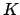be a number field of degree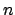. Then there areembeddings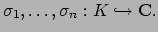Let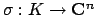be the product map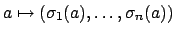. Let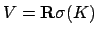be the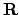-span of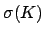inside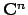.

Proposition 10.1.1   The-vector spacespanned by the imagehas dimension.

Proof. We prove this by showing that the image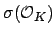is discrete. Ifwere not discrete it would contain elements all of whose coordinates are simultaneously arbitrarily small. The norm of an element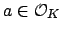is the product of the entries of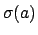, so the norms of nonzero elements of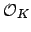would go to 0. This is a contradiction, since the norms of elements ofare integers.

The fact thatis discrete inimplies that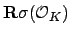has dimension equal to the rankof, as claimed. This last assertion is not obvious, and requires observing that if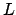if a free abelian group that is discrete in a real vector space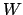and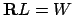, then the rank ofequals the dimension of. Here's why this is true. If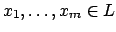are a basis for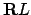, then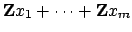has finite index in, since otherwise there would be infinitely many elements ofin a fundamental domain for, which would contradict discreteness of. Thus the rank ofis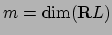, as claimed.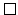Sinceis a lattice in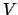, the volume of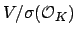is finite. Suppose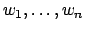is a basis for. Then if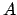is the matrix whose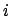th row is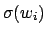, then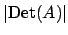is the volume of. (Take this determinant as the definition of the volume--we won't be using volume'' here except in a formal motivating way.)

Example 10.1.2   Let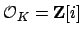be the ring of integers of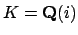. Then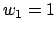,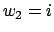is a basis for. The map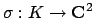is given by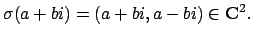The imageis spanned by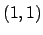and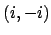. The volume determinant is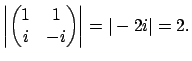Let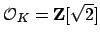be the ring of integers of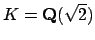. The map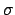is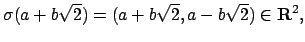and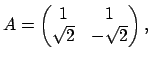which has determinant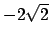, so the volume of the ring of integers is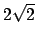.

As the above example illustrates, the volume of the ring of integers is not a great invariant of. For example, it need not be an integer. If we consider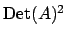instead, we obtain a number that is a well-defined integer which can be either positive or negative. In the next section we will do just this.

William Stein 2004-05-06